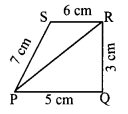# Samacheer Kalvi 8th Maths Solutions Term 1 Chapter 4 Geometry Intext Questions

Students can Download Maths Chapter 4 Geometry Intext Questions and Answers, Notes Pdf, Samacheer Kalvi 8th Maths Book Solutions Guide Pdf helps you to revise the complete Tamilnadu State Board New Syllabus and score more marks in your examinations.## Tamilnadu Samacheer Kalvi 8th Maths Solutions Term 1 Chapter 4 Geometry Intext Questions

Question 1.
The sum of the three angles of a triangle is ______
Solution:
1800

Question 2.
The exterior angle of a triangle is equal to the sum of the _______ angles opposite to it.
Solution:
interior

Question 3.
In a triangle, the sum of any two sides is ____ than the third side.
Solution:
greater

Question 4.
The difference between any two sides of a triangle is _______ than the third side.
Solution:
Smaller

Question 5.
Angles opposite to equal sides are ______ and vice-versa.
Solution:
Equal

Question 6.
The angles of a triangle are in the ratio 4 : 5 : 6
(i) Is it an acute, right or obtuse triangle?
(ii) Is it scalene, isosceles or equilateral?
Solution:
(i) Given the angles of a triangle are in the ratio 4 : 5 : 6 Sum of three angles of a
triangle = 180°.
Let the three angles 4x, 5x and 6x
4x + 5x + 6x = 180°
15x = 180° [∵ Vertically opposite angles are equal]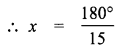∴ x = 12°
∴ The angles are 4x ⇒ 4 × 12 = 48°
5x ⇒ 5 × 12 = 60°
6x ⇒ 6 × 12 = 72°
∴ The angle of the triangle are 48°, 60°, 72°
∴ It is an acute angles triangle.

(ii) We know that the sides opposite to equal angles are equal.
Here all the three angles are different.
∴ The sides also different.
∴ The triangle is a scalene triangle.Question 7.
What is ∠A in the triangle ABC?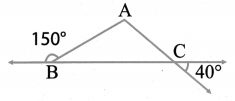Solution:
The exterior angle = sum of interior opposite angles.
∴ ∠A + ∠C = 150° in ∆ABC
But ∠C = 40° [∵ Vertically opposite angles are equal]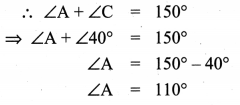Question 8.
Can a triangle have two supplementary angles? Why?
Solution:
Sum of three angles of a triangle is 180°.
∴ Sum of any two angles in a triangle will be less than 180°.
∴ A triangle cannot have two supplimentary angles.

Question 9.
________ shapes have the same shapes but different sizes.
Solution:
Similar

Question 10.
shapes are exactly the same in shape and size.
Solution:
Congruent

Exercise 4.1

Try these Page No. 99

Identify the pairs of shapes which are similar and congruent.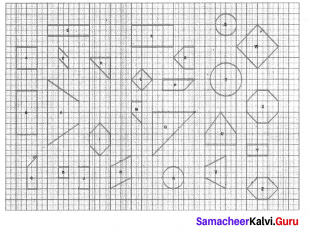Similar shapes:
(i) W and L
(ii) B and J
(iii) A and G
(iv) B and J
(v) B and Y
Congruent shapes:
(i) Z and I
(ii) J and Y
(iii) C and P You can find more.
(iv) B and K
(v) R and S
(vi) I and ZTry these Page No. 108

Question 1.
Match the following by their congruence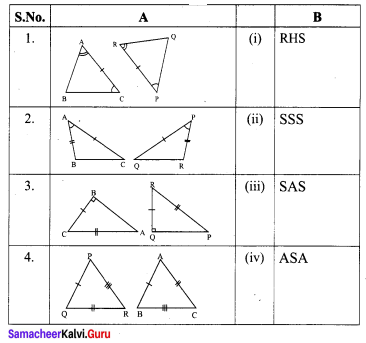Solution:
1 – (iv)
2 – (iii)
3 – (i)
4 – (ii)

Question 1.
In the figure, DA = DC and BA = BC. Are the triangles DBA and DBC congruent? Why?
Solution: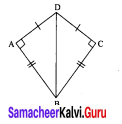AB = CB
DB = DB (common)
∆DBA ≅ ∆DBC [∵ By SSS Congruency]
Also RHS rule also bind here to say their congruency.

Exercise 4.3